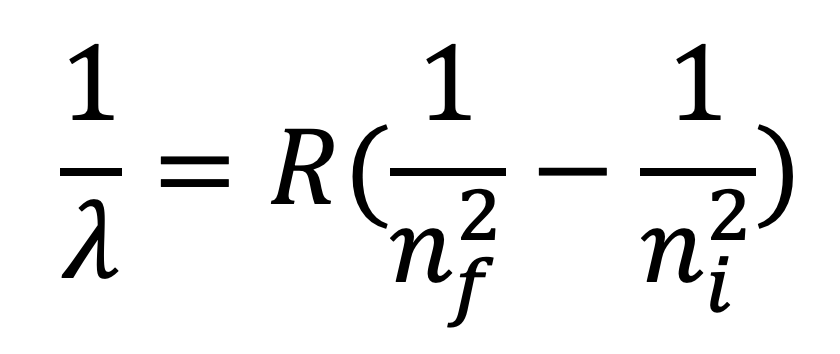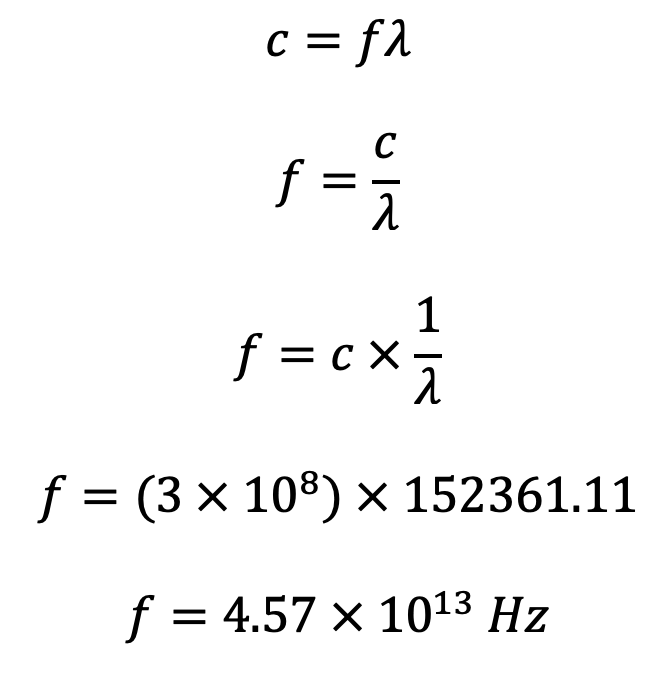# M8-7b: Rydberg's Equation

• ### relate qualitatively and quantitatively the quantised energy levels of the hydrogen atom and the law of conservation of energy to the line emission spectrum of hydrogen using:

- E = hf

- E = hc/λ

- Rydberg's equation

• Balmer's initial equation was first devised through empirically examining the lines in the hydrogen spectrum and creating a formula for them to fit in.
• Using Bohr's postulates and some manipulation of mathematics, Rydberg later was able to create the same formula which Balmer has derived.
• In this equation the Rydberg constant was introduced (R = 1.097x107 m-1) and is known as the Rydberg Equation:• Conservation of energy – Bohr postulated that an electron is a discrete orbit (energy level) does not emit energy. Therefore, when an electron returns to a lower energy level, it must emit the exact amount of energy it first absorbed to reach that energy level. In other words, the energy emitted by an electron during spectroscopy equals to the amount absorbed.where λ is the wavelength of emitted radiation, R is Rydberg’s constant (1.097x10m-1), nf and ni are the final and initial energy level during an electron’s transition respectively.

• Rydberg’s equation quantitatively determines the wavelength of photons in the Balmer series and could be used for other series and atoms.where Z is the number of protons in the atom/ion’s nucleus.

• However, the accuracy of prediction made by Rydberg’s equation rapidly decreases as the atom becomes larger. Hence, it is often only used for the hydrogen atom.

Practice Question 1

Hydrogen shows a large number of spectral lines, the visible ones being part of the Balmer series where nf = 2.

(a) Calculate the frequency of radiation emitted when an electron drops from ni = 3 to nf = 2. (2 marks)

(b) What type of radiation is emitted by the drop from ni = 3 to nf = 2? (1 mark)

Solution for Practice Question 1

(a)  Using Rydberg's equation:Since(b) The photon emitted will be in the visible light spectrum because it is the first transition of the Balmer Series. Specifically, it is within the red end of the visible spectrum.

Previous section: Bohr's and Rutherford's Atomic Models and their Limitations